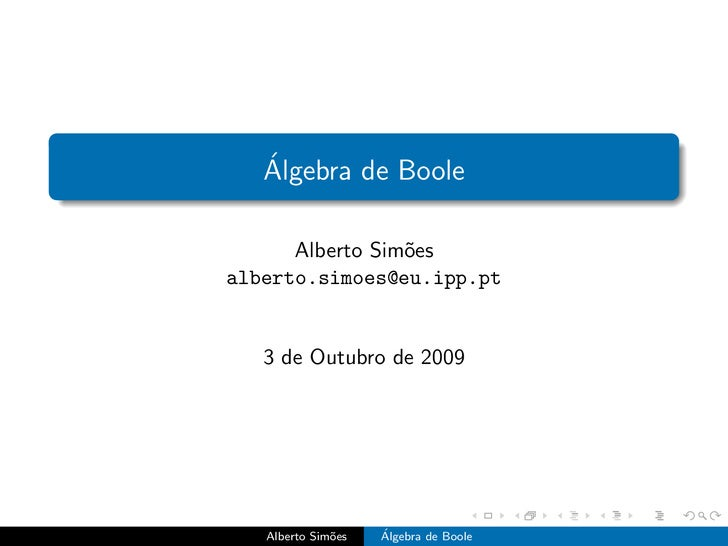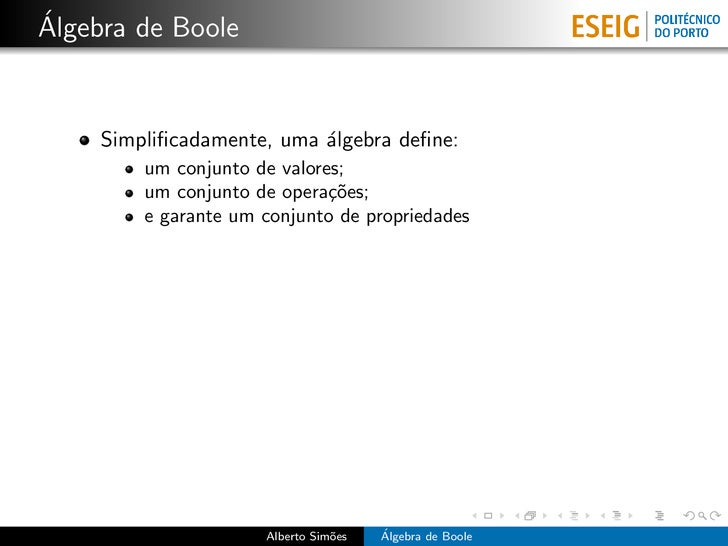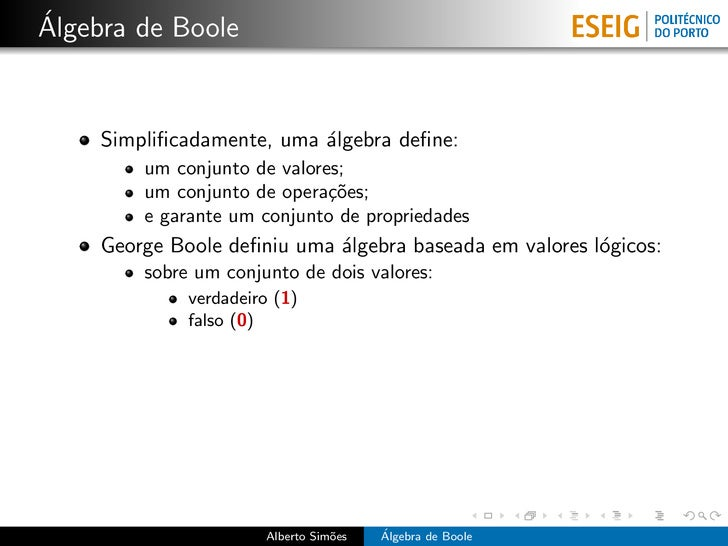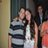Successfully reported this slideshow.Upcoming SlideShare
×

ofUpcoming SlideShare
Algebra De Boole
Next

Share

# Álgebra de Boole

Apresentação BÁSICA sobre álgebra de Boole e algumas noções de lógica de primeira ordem.

See all

See all

### Álgebra de Boole

1. 1. ´ Algebra de Boole Alberto Sim˜es o alberto.simoes@eu.ipp.pt 3 de Outubro de 2009 Alberto Sim˜es o ´ Algebra de Boole
2. 2. ´ Algebra de Boole Simpliﬁcadamente, uma ´lgebra deﬁne: a um conjunto de valores; um conjunto de opera¸˜es; co e garante um conjunto de propriedades Alberto Sim˜es o ´ Algebra de Boole
3. 3. ´ Algebra de Boole Simpliﬁcadamente, uma ´lgebra deﬁne: a um conjunto de valores; um conjunto de opera¸˜es; co e garante um conjunto de propriedades George Boole deﬁniu uma ´lgebra baseada em valores l´gicos: a o sobre um conjunto de dois valores: verdadeiro (1) falso (0) Alberto Sim˜es o ´ Algebra de Boole
4. 4. ´ Algebra de Boole Simpliﬁcadamente, uma ´lgebra deﬁne: a um conjunto de valores; um conjunto de opera¸˜es; co e garante um conjunto de propriedades George Boole deﬁniu uma ´lgebra baseada em valores l´gicos: a o sobre um conjunto de dois valores: verdadeiro (1) falso (0) com trˆs opera¸˜es b´sicas: e co a nega¸˜o (n˜o) ca a conjun¸˜o (e) ca disjun¸˜o (ou) ca Alberto Sim˜es o ´ Algebra de Boole
5. 5. ´ Algebra de Boole Nega¸˜o ca A nega¸˜o ´ habitualmente lida n˜o (not); ca e a A sua representa¸˜o matem´tica ´ ¬; ca a e Alberto Sim˜es o ´ Algebra de Boole
6. 6. ´ Algebra de Boole Nega¸˜o ca A nega¸˜o ´ habitualmente lida n˜o (not); ca e a A sua representa¸˜o matem´tica ´ ¬; ca a e x ¬x 0 1 1 0 Alberto Sim˜es o ´ Algebra de Boole
7. 7. ´ Algebra de Boole Nega¸˜o ca A nega¸˜o ´ habitualmente lida n˜o (not); ca e a A sua representa¸˜o matem´tica ´ ¬; ca a e x ¬x 0 1 1 0 ¬0 = 1 ¬1 = 0 Alberto Sim˜es o ´ Algebra de Boole
8. 8. ´ Algebra de Boole Conjun¸˜o ca A conjun¸˜o ´ habitualmente lida e (and); ca e A sua representa¸˜o matem´tica ´ ∧; ca a e Alberto Sim˜es o ´ Algebra de Boole
9. 9. ´ Algebra de Boole Conjun¸˜o ca A conjun¸˜o ´ habitualmente lida e (and); ca e A sua representa¸˜o matem´tica ´ ∧; ca a e ∧ 0 1 0 0 0 1 0 1 Alberto Sim˜es o ´ Algebra de Boole
10. 10. ´ Algebra de Boole Conjun¸˜o ca A conjun¸˜o ´ habitualmente lida e (and); ca e A sua representa¸˜o matem´tica ´ ∧; ca a e ∧ 0 1 0 0 0 1 0 1 1∧1 = 1 1∧0 = 0 0∧1 = 0 0∧0 = 0 Alberto Sim˜es o ´ Algebra de Boole
11. 11. ´ Algebra de Boole Disjun¸˜o ca A disjun¸˜o ´ habitualmente lida ou (or); ca e A sua representa¸˜o matem´tica ´ ∨; ca a e Alberto Sim˜es o ´ Algebra de Boole
12. 12. ´ Algebra de Boole Disjun¸˜o ca A disjun¸˜o ´ habitualmente lida ou (or); ca e A sua representa¸˜o matem´tica ´ ∨; ca a e ∨ 0 1 0 0 1 1 1 1 Alberto Sim˜es o ´ Algebra de Boole
13. 13. ´ Algebra de Boole Disjun¸˜o ca A disjun¸˜o ´ habitualmente lida ou (or); ca e A sua representa¸˜o matem´tica ´ ∨; ca a e ∨ 0 1 0 0 1 1 1 1 1∨1 = 1 1∨0 = 1 0∨1 = 1 0∨0 = 0 Alberto Sim˜es o ´ Algebra de Boole
14. 14. ´ Algebra de Boole Operadores Derivados Implica¸˜o: ca a ⇒ b = ¬(a ∧ ¬b) Ou exclusivo: a ⊕ b = (a ∨ b) ∧ ¬(a ∧ b) Equivalˆncia: e a ≡ b = ¬(a ⊕ b) Alberto Sim˜es o ´ Algebra de Boole
15. 15. ´ Algebra de Boole Implica¸˜o ca A implica¸˜o ´ habitualmente lida implica (implies); ca e A sua representa¸˜o matem´tica ´ ⇒; ca a e Alberto Sim˜es o ´ Algebra de Boole
16. 16. ´ Algebra de Boole Implica¸˜o ca A implica¸˜o ´ habitualmente lida implica (implies); ca e A sua representa¸˜o matem´tica ´ ⇒; ca a e ⇒ 0 1 0 1 1 1 0 1 Alberto Sim˜es o ´ Algebra de Boole
17. 17. ´ Algebra de Boole Implica¸˜o ca A implica¸˜o ´ habitualmente lida implica (implies); ca e A sua representa¸˜o matem´tica ´ ⇒; ca a e ⇒ 0 1 0 1 1 1 0 1 1⇒1 = 1 1⇒0 = 0 0⇒1 = 1 0⇒0 = 1 Alberto Sim˜es o ´ Algebra de Boole
18. 18. ´ Algebra de Boole Ou exclusivo O ou exclusivo ´ habitualmente denotado por xor; e a ⊕ b pode ser lido como a ou b, mas nunca os dois; N˜o tem uma representa¸˜o matem´tica oﬁcial; a ca a Nestes slides, ser´ usado o ⊕; a Alberto Sim˜es o ´ Algebra de Boole
19. 19. ´ Algebra de Boole Ou exclusivo O ou exclusivo ´ habitualmente denotado por xor; e a ⊕ b pode ser lido como a ou b, mas nunca os dois; N˜o tem uma representa¸˜o matem´tica oﬁcial; a ca a Nestes slides, ser´ usado o ⊕; a ⊕ 0 1 0 0 1 1 1 0 Alberto Sim˜es o ´ Algebra de Boole
20. 20. ´ Algebra de Boole Ou exclusivo O ou exclusivo ´ habitualmente denotado por xor; e a ⊕ b pode ser lido como a ou b, mas nunca os dois; N˜o tem uma representa¸˜o matem´tica oﬁcial; a ca a Nestes slides, ser´ usado o ⊕; a ⊕ 0 1 0 0 1 1 1 0 1⊕1 = 0 1⊕0 = 1 0⊕1 = 1 0⊕0 = 0 Alberto Sim˜es o ´ Algebra de Boole
21. 21. ´ Algebra de Boole Equivalˆncia e A equivalˆncia ´ habitualmente lida equivale a; e e A sua representa¸˜o matem´tica ´ ≡; ca a e Alberto Sim˜es o ´ Algebra de Boole
22. 22. ´ Algebra de Boole Equivalˆncia e A equivalˆncia ´ habitualmente lida equivale a; e e A sua representa¸˜o matem´tica ´ ≡; ca a e ≡ 0 1 0 1 0 1 0 1 Alberto Sim˜es o ´ Algebra de Boole
23. 23. ´ Algebra de Boole Equivalˆncia e A equivalˆncia ´ habitualmente lida equivale a; e e A sua representa¸˜o matem´tica ´ ≡; ca a e ≡ 0 1 0 1 0 1 0 1 1≡1 = 1 1≡0 = 0 0≡1 = 0 0≡0 = 1 Alberto Sim˜es o ´ Algebra de Boole
24. 24. ´ Algebra de Boole Leis b´sicas a Associatividade da disjun¸˜o e conjun¸˜o: ca ca a ∧ (b ∧ c) = (a ∧ b) ∧ c a ∨ (b ∨ c) = (a ∨ b) ∨ c Alberto Sim˜es o ´ Algebra de Boole
25. 25. ´ Algebra de Boole Leis b´sicas a Associatividade da disjun¸˜o e conjun¸˜o: ca ca a ∧ (b ∧ c) = (a ∧ b) ∧ c a ∨ (b ∨ c) = (a ∨ b) ∨ c Comutatividade da disjun¸˜o e conjun¸˜o: ca ca a∧b =b∧a a∨b =b∨a Alberto Sim˜es o ´ Algebra de Boole
26. 26. ´ Algebra de Boole Leis b´sicas a Associatividade da disjun¸˜o e conjun¸˜o: ca ca a ∧ (b ∧ c) = (a ∧ b) ∧ c a ∨ (b ∨ c) = (a ∨ b) ∨ c Comutatividade da disjun¸˜o e conjun¸˜o: ca ca a∧b =b∧a a∨b =b∨a Distributividade: a ∧ (b ∨ c) = (a ∧ b) ∨ (a ∧ c) a ∨ (b ∧ c) = (a ∨ b) ∧ (a ∨ c) Alberto Sim˜es o ´ Algebra de Boole
27. 27. ´ Algebra de Boole Leis b´sicas a Dupla Nega¸˜o ca ¬¬a = a Alberto Sim˜es o ´ Algebra de Boole
28. 28. ´ Algebra de Boole Leis b´sicas a Dupla Nega¸˜o ca ¬¬a = a De Morgan ¬(a ∧ b) = ¬a ∨ ¬b ¬(a ∨ b) = ¬a ∧ ¬b Alberto Sim˜es o ´ Algebra de Boole
29. 29. ´ Algebra de Boole Leis b´sicas a Dupla Nega¸˜o ca ¬¬a = a De Morgan ¬(a ∧ b) = ¬a ∨ ¬b ¬(a ∨ b) = ¬a ∧ ¬b Mais em http: //en.wikipedia.org/wiki/Elementary_Boolean_algebra Alberto Sim˜es o ´ Algebra de Boole
•#### liliameirlelles

Oct. 10, 2017
•#### Mateus2898

Mar. 12, 2013

Total views

5,145

On Slideshare

0

From embeds

0

Number of embeds

65

160

Shares

0